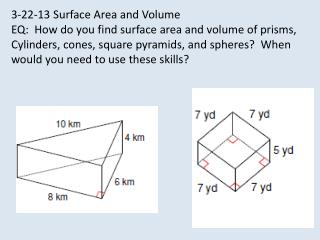# 3-22-13 Surface Area and Volume EQ: How do you find surface area and volume of prisms, - PowerPoint PPT PresentationDownload Presentation3-22-13 Surface Area and Volume EQ: How do you find surface area and volume of prisms,

3-22-13 Surface Area and Volume EQ: How do you find surface area and volume of prisms,Download Presentation## 3-22-13 Surface Area and Volume EQ: How do you find surface area and volume of prisms,

- - - - - - - - - - - - - - - - - - - - - - - - - - - E N D - - - - - - - - - - - - - - - - - - - - - - - - - - -
##### Presentation Transcript

1. 3-22-13 Surface Area and Volume EQ: How do you find surface area and volume of prisms, Cylinders, cones, square pyramids, and spheres? When would you need to use these skills?

3. Math 7+ -Check Triangular Prism Handout Complete textbook page 555-556 tonight. Math 7 - Check handout given on Wednesday. Return quiz paper and review formulas. Finish work on formulas.

4. FORMULAS The fold crease Foldable Reopen the fold. *Draw each shape *Write the general formula for surface area of a rectangular prism. *Write the general formula for volume of A rectangular prism Rectangular Prism Triangular Prism Square Pyramid Cylinder cone

5. RECTANGULAR PRISM What are the steps to finding the surface area of a rectangular prism? Volume? SA = ph + 2B Remember the smiley face method Volume = Bh = lwh *base is a rectangle

6. CYLINDER 10 Diameter = 12 Volume = Bh = πr2h Surface Area = ph + 2B =πdh+ πr2 B = area of the base

7. How do you find the volume of a triangular prism? Surface area? SA = ph + 2B (s+s+s)h + 2(bh) 2 *Base is a triangle Identify the height of the prism. V= Bh =(bh)H 2 http://www.mrmaisonet.com/index.php?/Volume-Video/Volume-Of-A-Triangular-Prism.html

9. http://www.brightstorm.com/math/geometry/area/surface-area-of-cones/http://www.brightstorm.com/math/geometry/area/surface-area-of-cones/ http://www.brightstorm.com/math/geometry/volume/volume-of-cones/

10. SphereVolume = 4/3 πr3Surface Area = 4πr2 http://www.mrmaisonet.com/index.php?/Volume-Video/Volume-Of-A-Sphere.html

11. Math 7+ Complete workbook pages 175 and 176

12. Jennie purchased a box of crackers from the deli. The box is in the shape of a triangular prism. The height of the triangle is 10 cm. Find the surface area of the cracker box. 15 cm 15 cm 30 cm 18 cm

13. Juan covered a box with stick-backed decorating paper. The paper costs \$.07 cents per square inch. How much money will Juan need to spend on paper? Height = 9 inches Width = 3 inches Length = 7 inches

14. Math 7+ EXIT TICKET Surface Area: Pyramids, Cones, and Spheres Find the surface area of each space figure. Round to the nearest whole unit. 1. a square pyramid with base edge 80 m and slant height 100 m 2. a cone with slant height 22 cm and radius 7 cm 3. a sphere with radius 12 cm 22,400 m2 about 637 cm2 about 1,809 cm2 10-6

15. Summary: How will you remember the difference between surface area and volume? What will you do to learn the formulas? M7+ -Homework: Textbook pages 555-556, #1-14 M7 Homework: